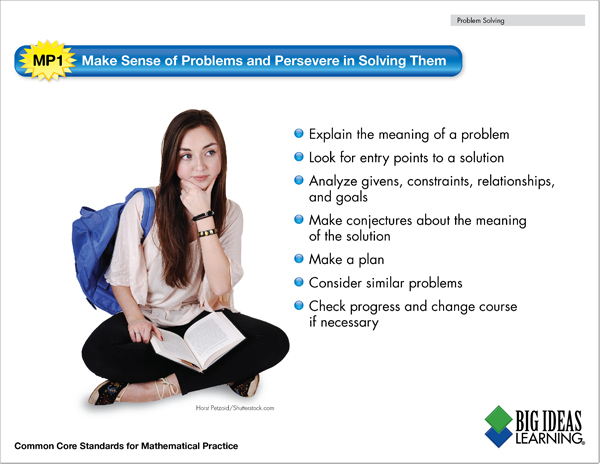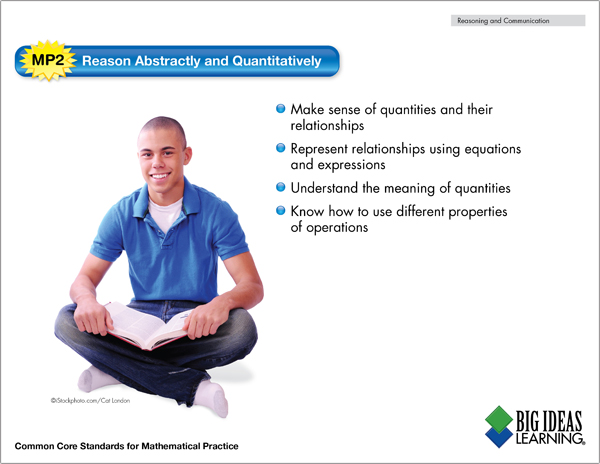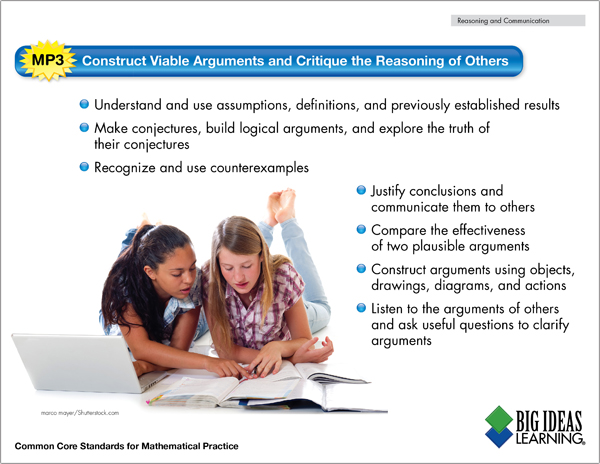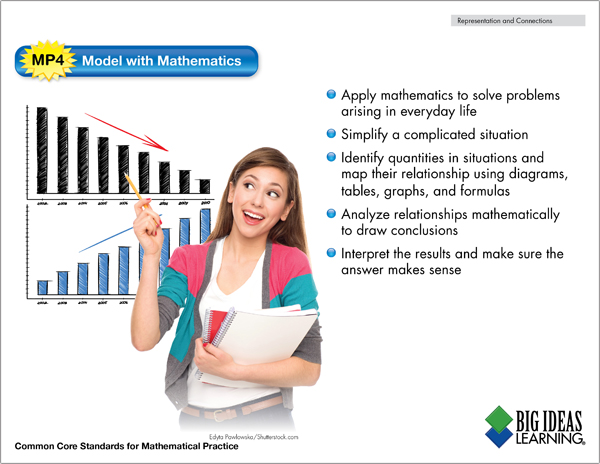Classroom Posters: Mathematical Practices #1-4

Have you started thinking about your classroom setup for the upcoming school year?

Big Ideas Math has another great set of resources for your classroom! Inspire your students to think strategically and apply a wide range of math strategies with our Mathematical Practice posters.

Use the PDF download link below each image to print the poster. Then the rest is up to you! Laminate each one and create a display wall in your classroom, or use them as a resource in cooperative groups as students are solving problems.

Check back on the Big Ideas Math blog next week for classroom posters of Mathematical Practices #5-8!

Mathematical Practice 1: Make Sense of Problems and Persevere in Solving ThemDownload the full poster: Mathematical Practice 1 Poster (PDF)

Mathematical Practice 2: Reason Abstractly and QuantitativelyDownload the full poster: Mathematical Practice 2 Poster (PDF)

Mathematical Practice 3: Construct Viable Arguments and Critique the Reasoning of OthersDownload the full poster: Mathematical Practice 3 Poster (PDF)

Mathematical Practice 4: Model with MathematicsDownload the full poster: Mathematical Practice 4 Poster (PDF)